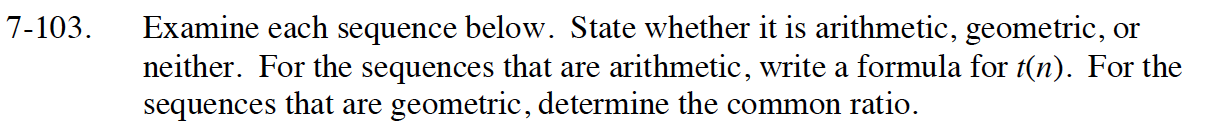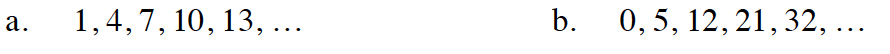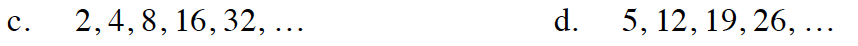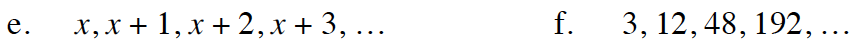Home > INT1 > Chapter Ch7 > Lesson 7.2.2 > Problem7-103

7-103.
1. Examine each sequence below. State whether it is arithmetic, geometric, or neither. For the sequences that are arithmetic, write a formula for t(n). For the sequences that are geometric, determine the common ratio. Homework Help ✎

2.  a. 1, 4, 7, 10, 13, … b. 0, 5, 12, 21, 32, … c. 2, 4, 8, 16, 32, … d. 5, 12, 19, 26, … e. x, x + 1, x + 2, x + 3, … f. 3, 12, 48, 192, …The sequence is neither arithmetic nor geometric.The sequence is geometric.

r = 2

Do the terms in the sequence increase by adding a constant amount or multiplying by a constant?See part (d).

The sequence is arithmetic.

d = 1

See part (d).